# Linear Equations & Systems: Tutoring Solution Chapter Exam

Exam Instructions:

Choose your answers to the questions and click 'Next' to see the next set of questions. You can skip questions if you would like and come back to them later with the yellow "Go To First Skipped Question" button. When you have completed the practice exam, a green submit button will appear. Click it to see your results. Good luck!

### Page 1

#### Question 3 3. Which variable in which equation needs to be eliminated in order to solve this system using Gaussian elimination?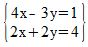#### Question 5 5. Look at the graph. What is the solution to the system of equations?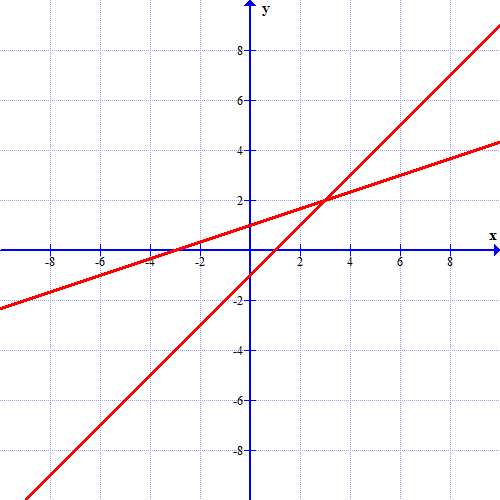### Page 3

#### Question 12 12. Solve the following system of equations.#### Question 13 13. What can you do to eliminate the x in the second equation?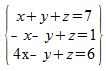### Page 4

#### Question 20 20. What is the solution to the system of equations shown in the graph below?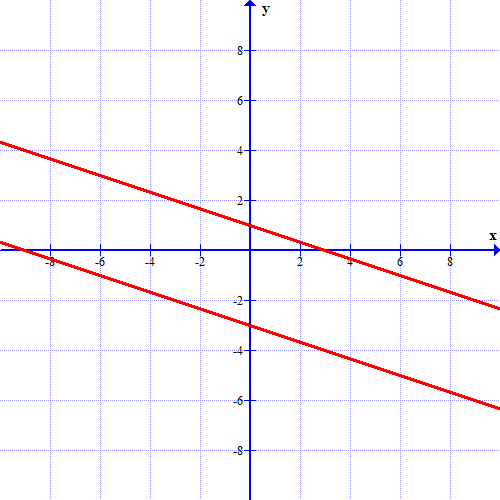### Page 6

#### Question 30 30. What is the equation of the graph below?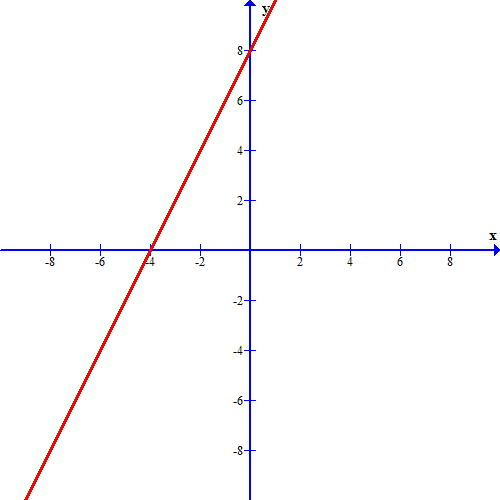#### Linear Equations & Systems: Tutoring Solution Chapter Exam Instructions

Choose your answers to the questions and click 'Next' to see the next set of questions. You can skip questions if you would like and come back to them later with the yellow "Go To First Skipped Question" button. When you have completed the practice exam, a green submit button will appear. Click it to see your results. Good luck!

Support